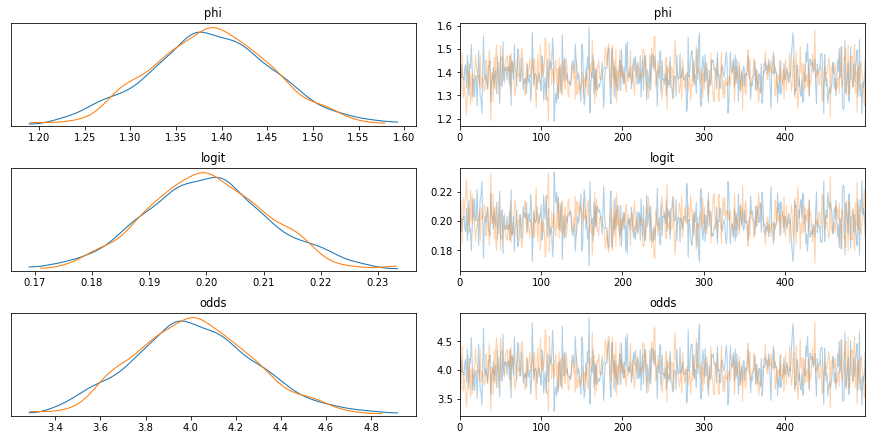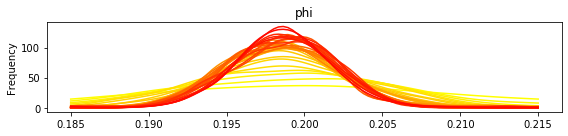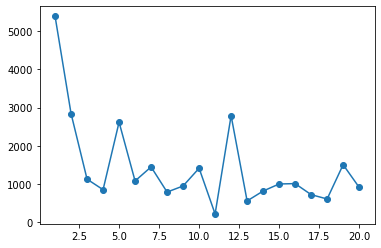In :
import pymc3 as pm
from pymc3.distributions import Interpolated
import theano
import numpy as np
%matplotlib inline
import matplotlib.pyplot as plt

#see: https://docs.pymc.io/notebooks/updating_priors.html


# Generate some data:¶

This is for a hierarchical model to measure the proportion of some event. It generates about 1 to 10 samples of binary 0 or 1 measurements, where 1's (the event) occur 20% of the time.

There will be some variability. So the goal is to measure the proportion of 1's while modeling each instance individually and sharing overall mean probability --> i.e. a hierarchical model

We thus expect the probability to be ~20%, and/or the odds to be ~1/4.

### The data:¶

In :
#generate data:
#Creating a synthetic dataset:
data = list()

#probability of 0 or 1:
probs = [0.8, 0.2]
#take measurements for 500 instances:
def gen_data(N):
for j in range(N):

observations = list()
#all instances must have at least one measurement:
observations.append(np.random.choice([0,1], p=probs))
#now take several more measurements for this instance:
while np.random.uniform(0,1)>0.2:
observations.append(np.random.choice([0,1], p=probs))
data.append(observations)

#parse for input to pymc3:
num_measurements = [len(i) for i in data]
num_events = [sum(i) for i in data]
return np.array(num_measurements), np.array(num_events)

N=500
num_measurements, num_events = gen_data(N)

#num_measurements = theano.shared(num_measurements, name='num_measurements')
#num_events = theano.shared(num_events, name='num_events')


### The model:¶

The group parameter (phi) is measuring the log probability of the event. This can be transformed into a probability by a logit (logit) and then to odds (odds). The instance parameters are beta distributions, with parameters 'a' and 'b' determined by the odds, i.e. odds of 1:odds.

Likelihood is measured by a binomial, where you use the probability determined by the priors above along with the number of measurements and the sum of measurements for each instance as the observations.

In :
traces = []
#pymc3 gear:
with pm.Model() as model:
#group parameter:
phi = pm.Normal('phi', mu=0, sigma=1.0)
#probability:
logit = pm.Deterministic('logit', 1 / (1 + theano.tensor.exp(phi)))
odds = pm.Deterministic('odds', (1-logit)/logit)
#instance parameters:
thetas = pm.Beta('thetas', alpha=1, beta=odds, shape=N)
#likelihood:
y = pm.Binomial('y', n=num_measurements, p=thetas, observed=num_events)

trace = pm.sample(tune=500, draws=500)
traces.append(trace)

Auto-assigning NUTS sampler...
Initializing NUTS using jitter+adapt_diag...
Multiprocess sampling (2 chains in 2 jobs)
NUTS: [thetas, phi]

100.00% [2000/2000 00:07<00:00 Sampling 2 chains, 0 divergences]
In :
pm.traceplot(trace, var_names=['phi', 'logit', 'odds'])

/Users/lmar3213/miniconda3/envs/ppl/lib/python3.8/site-packages/arviz/plots/backends/matplotlib/distplot.py:36: UserWarning: Argument backend_kwargs has not effect in matplotlib.plot_distSupplied value won't be used
warnings.warn(
/Users/lmar3213/miniconda3/envs/ppl/lib/python3.8/site-packages/arviz/plots/backends/matplotlib/distplot.py:36: UserWarning: Argument backend_kwargs has not effect in matplotlib.plot_distSupplied value won't be used
warnings.warn(
/Users/lmar3213/miniconda3/envs/ppl/lib/python3.8/site-packages/arviz/plots/backends/matplotlib/distplot.py:36: UserWarning: Argument backend_kwargs has not effect in matplotlib.plot_distSupplied value won't be used
warnings.warn(

Out:
array([[<matplotlib.axes._subplots.AxesSubplot object at 0x1335d98b0>,
<matplotlib.axes._subplots.AxesSubplot object at 0x128b7d640>],
[<matplotlib.axes._subplots.AxesSubplot object at 0x137d66040>,
<matplotlib.axes._subplots.AxesSubplot object at 0x13365d400>],
[<matplotlib.axes._subplots.AxesSubplot object at 0x12a074eb0>,
<matplotlib.axes._subplots.AxesSubplot object at 0x132852580>]],
dtype=object)In :
from scipy import stats
##This will generate a 'distribution' empirically from the posterior of a given variable from a run.
def from_posterior(param, samples):
smin, smax = np.min(samples), np.max(samples)
width = smax - smin
x = np.linspace(smin, smax, 100)
y = stats.gaussian_kde(samples)(x)

# what was never sampled should have a small probability but not 0,
# so we'll extend the domain and use linear approximation of density on it
x = np.concatenate([[x - 3 * width], x, [x[-1] + 3 * width]])
y = np.concatenate([, y, ])
return Interpolated(param, x, y)

In :
for _ in range(10):
print('iteration:', _)
num_measurements, num_events

model = pm.Model()
with model:
#group parameter:
phi = from_posterior('phi', trace['phi'])

#probability:
logit = pm.Deterministic('logit', 1 / (1 + theano.tensor.exp(phi)))
odds = pm.Deterministic('odds', (1-logit)/logit)
#instance parameters:
thetas = pm.Beta('thetas', alpha=1, beta=odds, shape=N)
#likelihood:
y = pm.Binomial('y', n=num_measurements, p=thetas, observed=num_events)

trace = pm.sample(tune=500, draws=500)
traces.append(trace)

iteration: 0

Auto-assigning NUTS sampler...
Initializing NUTS using jitter+adapt_diag...
Multiprocess sampling (2 chains in 2 jobs)
NUTS: [thetas, phi]

100.00% [2000/2000 00:08<00:00 Sampling 2 chains, 0 divergences]
iteration: 1

Auto-assigning NUTS sampler...
Initializing NUTS using jitter+adapt_diag...
Multiprocess sampling (2 chains in 2 jobs)
NUTS: [thetas, phi]

100.00% [2000/2000 00:06<00:00 Sampling 2 chains, 0 divergences]
iteration: 2

Auto-assigning NUTS sampler...
Initializing NUTS using jitter+adapt_diag...
Multiprocess sampling (2 chains in 2 jobs)
NUTS: [thetas, phi]

100.00% [2000/2000 00:07<00:00 Sampling 2 chains, 0 divergences]
iteration: 3

Auto-assigning NUTS sampler...
Initializing NUTS using jitter+adapt_diag...
Multiprocess sampling (2 chains in 2 jobs)
NUTS: [thetas, phi]

100.00% [2000/2000 00:08<00:00 Sampling 2 chains, 0 divergences]
iteration: 4

Auto-assigning NUTS sampler...
Initializing NUTS using jitter+adapt_diag...
Multiprocess sampling (2 chains in 2 jobs)
NUTS: [thetas, phi]

100.00% [2000/2000 00:07<00:00 Sampling 2 chains, 0 divergences]
iteration: 5

Auto-assigning NUTS sampler...
Initializing NUTS using jitter+adapt_diag...
Multiprocess sampling (2 chains in 2 jobs)
NUTS: [thetas, phi]

100.00% [2000/2000 00:13<00:00 Sampling 2 chains, 0 divergences]
iteration: 6

Auto-assigning NUTS sampler...
Initializing NUTS using jitter+adapt_diag...
Multiprocess sampling (2 chains in 2 jobs)
NUTS: [thetas, phi]

100.00% [2000/2000 00:08<00:00 Sampling 2 chains, 0 divergences]
iteration: 7

Auto-assigning NUTS sampler...
Initializing NUTS using jitter+adapt_diag...
Multiprocess sampling (2 chains in 2 jobs)
NUTS: [thetas, phi]

100.00% [2000/2000 00:08<00:00 Sampling 2 chains, 0 divergences]
The estimated number of effective samples is smaller than 200 for some parameters.

iteration: 8

Auto-assigning NUTS sampler...
Initializing NUTS using jitter+adapt_diag...
Multiprocess sampling (2 chains in 2 jobs)
NUTS: [thetas, phi]

100.00% [2000/2000 00:09<00:00 Sampling 2 chains, 0 divergences]
iteration: 9

Auto-assigning NUTS sampler...
Initializing NUTS using jitter+adapt_diag...
Multiprocess sampling (2 chains in 2 jobs)
NUTS: [thetas, phi]

100.00% [2000/2000 00:08<00:00 Sampling 2 chains, 0 divergences]
In :
import matplotlib as mpl
print('Posterior distributions after ' + str(len(traces)) + ' iterations.')
cmap = mpl.cm.autumn
for param in ['phi']:
plt.figure(figsize=(8, 2))
for update_i, tr in enumerate(traces):
samples = tr[param]
#smin, smax = np.min(samples), np.max(samples)
p = 1 / (1+np.exp(samples))
smin, smax = np.min(p), np.max(p)
#x = np.linspace(smin, smax, 100)
x = np.linspace(0.185, 0.215, 100)
y = stats.gaussian_kde(p)(x)
plt.plot(x, y, color=cmap(1 - update_i / len(traces)))

plt.ylabel('Frequency')
plt.title(param)

plt.tight_layout();

Posterior distributions after 31 iterations.In :
pdfs = list()

for param in ['phi']:
for update_i, tr in enumerate(traces):
samples = tr[param]
p = 1 / (1+np.exp(samples))
smin, smax = np.min(p), np.max(p)
#x = np.linspace(smin, smax, 100)
x = np.linspace(0.185, 0.215, 100)
y = stats.gaussian_kde(p)(x)
pdfs.append(y)

In :
rmses = list()
for count, i in enumerate(range(1, 21)):
rmse = ((pdfs[i]-pdfs[i-1])**2).sum()
rmses.append(rmse)

plt.scatter(range(1,21),rmses)
plt.plot(range(1,21),rmses)

Out:
[<matplotlib.lines.Line2D at 0x14bebecd0>]In [ ]: